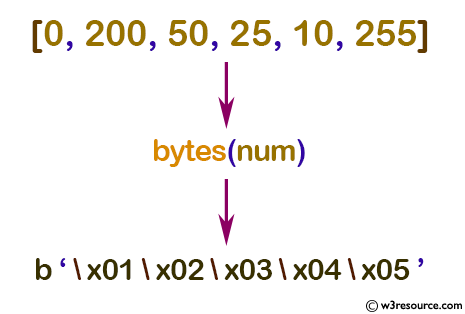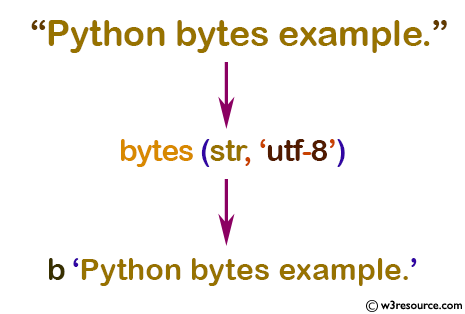# Python: bytes() function

## bytes() function

The bytes() function is used to get a new 'bytes' object.

Note: bytes is an immutable version of bytearray – it has the same non-mutating methods and the same indexing and slicing behavior.

Accordingly, constructor arguments are interpreted as for bytearray()

Syntax:

```bytes([source[, encoding[, errors]]])
```Version:

(Python 3.2.5)

Return value:

Return a new 'bytes' object, which is an immutable sequence of integers in the range 0 <= x < 256.

Example: Convert iterable list to bytes

``````num = [0, 200, 50, 25, 10, 255]

a = bytes(num)
print(a)
``````

Output:

```b'\x01\x02\x03\x04\x05'
```

Pictorial Presentation:Example: Create a byte of given integer size

``````s = 10

a = bytes(s)
print(a)
``````

Output:

```b'\x00\x00\x00\x00\x00\x00\x00\x00\x00\x00'
```

Example: Convert string to bytes

``````str = "Python bytes example."

# string with encoding 'utf-8'
a = bytes(str, 'utf-8')
print(a)
``````

Output:

```b'Python bytes example.'
```

Pictorial Presentation:Python Code Editor:

Previous: bytearray()
Next: callable()

Test your Python skills with w3resource's quiz

﻿

## Python: Tips of the Day

How do I check if a list is empty?

For example, if passed the following:

```a = []
if not a:
print("List is empty")
```

Ref: https://bit.ly/2A4JXx9